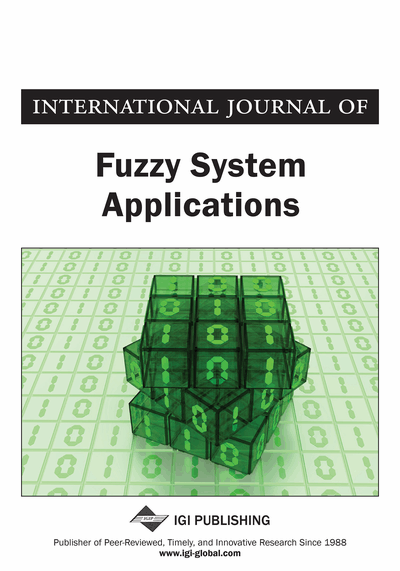# Intuitionistic Fuzzy Distance Based TOPSIS Method and Application to MADM

Jiangxia Nan (School of Mathematics and Computing Science, Guilin University of Electronic Technology, Guilin, China), Ting Wang (School of Mathematics and Computing Science, Guilin University of Electronic Technology, Guilin, China), and Jingjing An (School of Mathematics and Computing Science, Guilin University of Electronic Technology, Guilin, China)
DOI: 10.4018/IJFSA.2016010103
Available
\$29.50
No Current Special Offers

## Abstract

In this paper, an intuitionistic fuzzy (IF) distance measure between two triangular intuitionistic fuzzy numbers (TIFNs) is developed. The metric properties of the proposed IF distance measure are also studied. Then, based on this IF distance, an extended TOPSIS is developed to solve multi-attribute decision making (MADM) problems with the ratings of alternatives on attributes of TIFNs. In this methodology, the IF distances between each alternative and the TIFN positive ideal-solution are calculated as well as the TIFN negative ideal-solution. Then the relative closeness degrees obtained of each alternative to the TIFN positive ideal solution are TIFNs. Based on the ranking methods of TIFNs the alternatives are ranked. A numerical example is examined to the validity and practicability of the method proposed in this paper.
Article Preview
Top

## 1. Introduction

In the application of fuzzy numbers, distance measures between fuzzy numbers play a very important role. Thus some researchers have focused their attention to compute the distances between fuzzy numbers (Cheng, 1998; Chakraborty, 2006; Guha & Chakraborty, 2010; Tran & Duckstein, 2002; Voxman, 1998; Xu & Li, 2001). Atanassov (1999) proposed the intuitionistic fuzzy (IF) sets, which is an extension of the fuzzy set and it is better than fuzzy set in dealing with vagueness and uncertainty of more flexible and practical. Based on the study of distance measures between fuzzy numbers, some researchers studied the distance between IF sets (Grzegorzewski, 2004; Szmidt & Kacprzyk, 2000; Wang & Xin, 2005). As a specialization of IF-set, the concept of intuitionistic fuzzy numbers (IFNs) can express information more abundant and flexible than the fuzzy number. However, there is very little investigation on distance measure between IFNs. Grzegorzewski (2003) studied the distance of IFNs, which is expressed as crisp values. It is unreasonable, the reason is that from a viewpoint of logic, a distance measure between IFNs should be an IFN. In other word, the uncertainty or hesitation or lack of knowledge presented in defining IFN should inherently be involved in their corresponding distance measures. Guha and Chakraborty (2010) studied the distance measure between two IFNs, which is IFN. In this paper, extending the fuzzy distance given by Tran & Duckstein (2002), a new IF distance measure between two triangular intuitionistic fuzzy numbers (TIFNs) defined by Li (2010) is proposed based on interval difference. Here the distance between two TIFNs is also TIFN.

The TOPSIS method is an important method for MADM problems. Some researchers studied TOPSIS with IF information (Zhang & Xu, 2015; Chen, 2015; Li & Nan, 2011; Li, 2010, 2012). The basic premise of the TOPSIS is that the chosen alternative should be the shortest distance to the positive ideal solution, and the longest distance from the negative ideal solution simultaneously. Thus, the distance measure plays an important role in TOPSIS method. However, these distance measures in existed TOPSIS were expressed as crisp values, which is unreasonable. So far as we know, there is no investigation on using the IF distance measure in TOPSIS to solve MADM with TIFNs. Thus, based on the new IF distance measure defined in this paper, the TOPSIS is extended to solve MADM problems in which the ratings of alternatives on attributes of TIFNs. The distances of each alternative from the TIFNs positive ideal solution, the TIFNs negative ideal solution and the relative closeness degrees of each alternative to the TIFNs positive ideal solution are calculated and they are still TIFNs. Then according to the ranking method of the weighted value-indices and weighted ambiguity-indices of TIFNs given by Li et al. (2010), the alternatives are ranked.

## Complete Article List

Search this Journal:
Reset
Volume 12: 1 Issue (2023)
Volume 11: 4 Issues (2022)
Volume 10: 4 Issues (2021)
Volume 9: 4 Issues (2020)
Volume 8: 4 Issues (2019)
Volume 7: 4 Issues (2018)
Volume 6: 4 Issues (2017)
Volume 5: 4 Issues (2016)
Volume 4: 4 Issues (2015)
Volume 3: 4 Issues (2013)
Volume 2: 4 Issues (2012)
Volume 1: 4 Issues (2011)
View Complete Journal Contents Listing